# Competitive Lotka–Volterra Model

## Lotka–Volterra Equations

$\frac{dx_1}{dt}=r_1x_1(1-\frac{x_1 + \alpha_{12}x_2}{K_1})$ $\frac{dx_2}{dt}=r_2x_2(1-\frac{x_2 + \alpha_{21}x_1}{K_2})$

## Case 1: Stable Equilibrium (with random noises)

$K_1 < \frac{K_2}{\alpha_{21}}$ $K_2 < \frac{K_1}{\alpha_{12}}$

Set $$K_1=100$$, $$K_2=100$$, $$\alpha_{12}=0.6$$, $$\alpha_{21}=0.6$$,

$$r_1 = 0.1$$, $$r_2 = 0.1$$,

and initial population size: $$x_1=5$$, $$x_2=10$$.

### Time series

plot_DE(cp_lotka(r1=0.1,r2=0.1, a12=0.6,a21=0.6, K1=100,K2=100, x1_int=5, x2_int=10))
##  62.5 62.5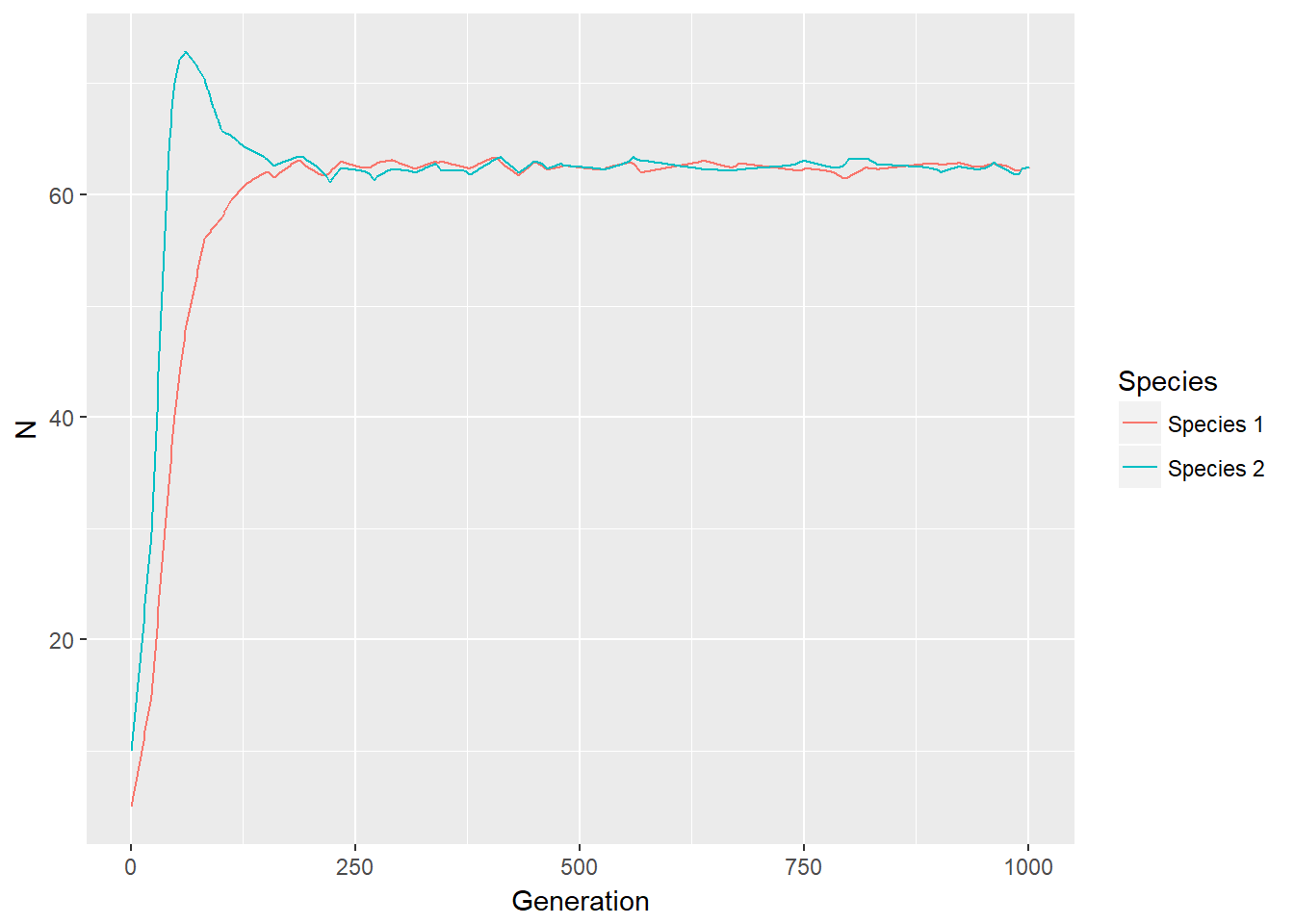### Phase portrait

p <- c(r1=0.1,r2=0.1, a12=0.6,a21=0.6, K1=100,K2=100) # p is a named vector of parameters
s <- c(x1=5,x2=10) # s is the state
plane(tstep=0.5,portrait=T, xmax=150,ymax=150)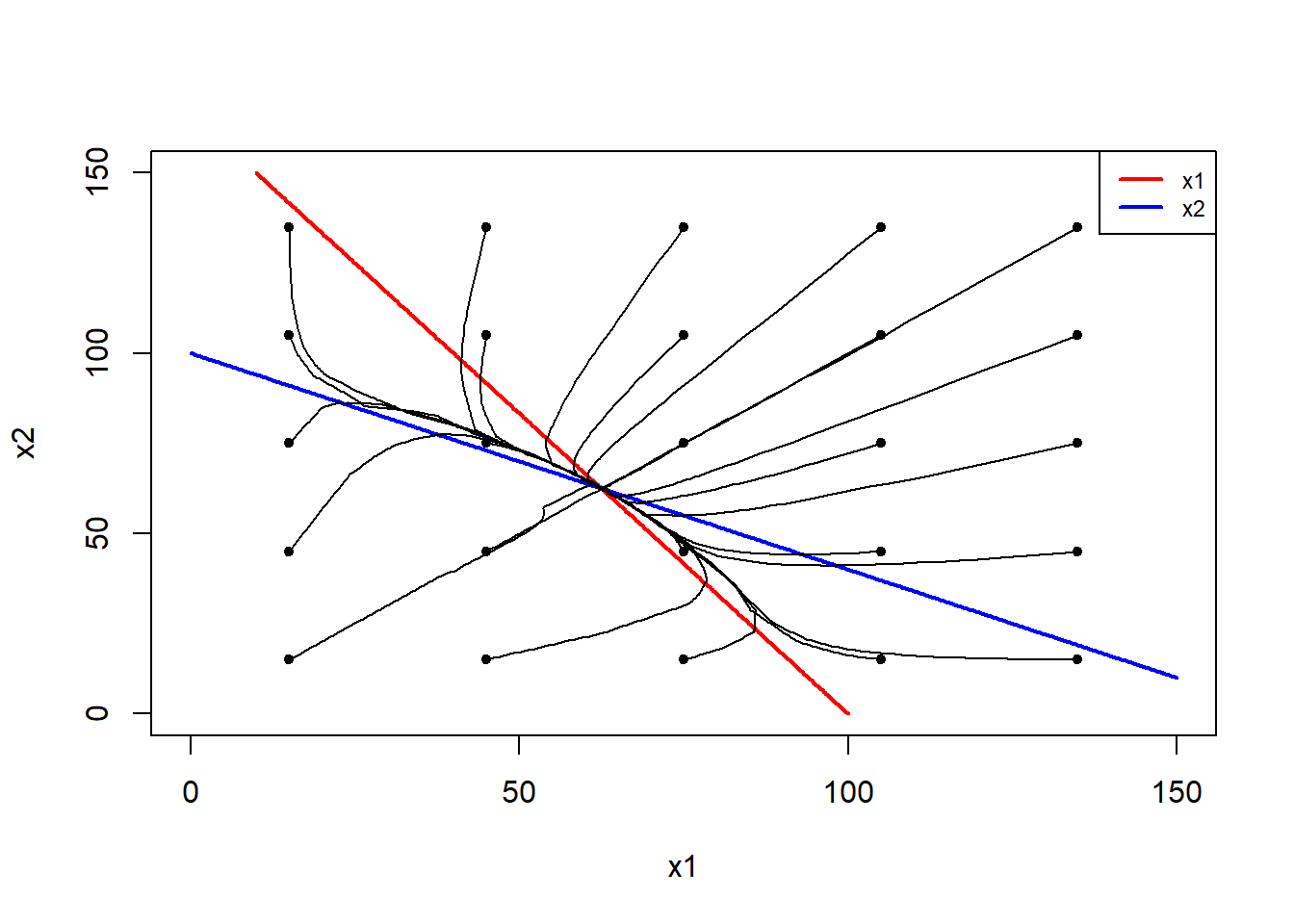## Case 2: Unstable Equilibrium (with random noises)

$K_1 > \frac{K_2}{\alpha_{21}}$ $K_2 > \frac{K_1}{\alpha_{12}}$ Set $$K_1=100$$, $$K_2=100$$, $$\alpha_{12}=1.2$$, $$\alpha_{21}=1.2$$,

and same initial population size for the two species: $$x_1=10$$, $$x_2=10$$.

### Time series: species coexist

Set $$r_1 = 0.1$$, $$r_2 = 0.1$$

plot_DE(cp_lotka(r1=0.1,r2=0.1, a12=1.2,a21=1.2, K1=100,K2=100, x1_int=10, x2_int=10))
##  45.45455 45.45455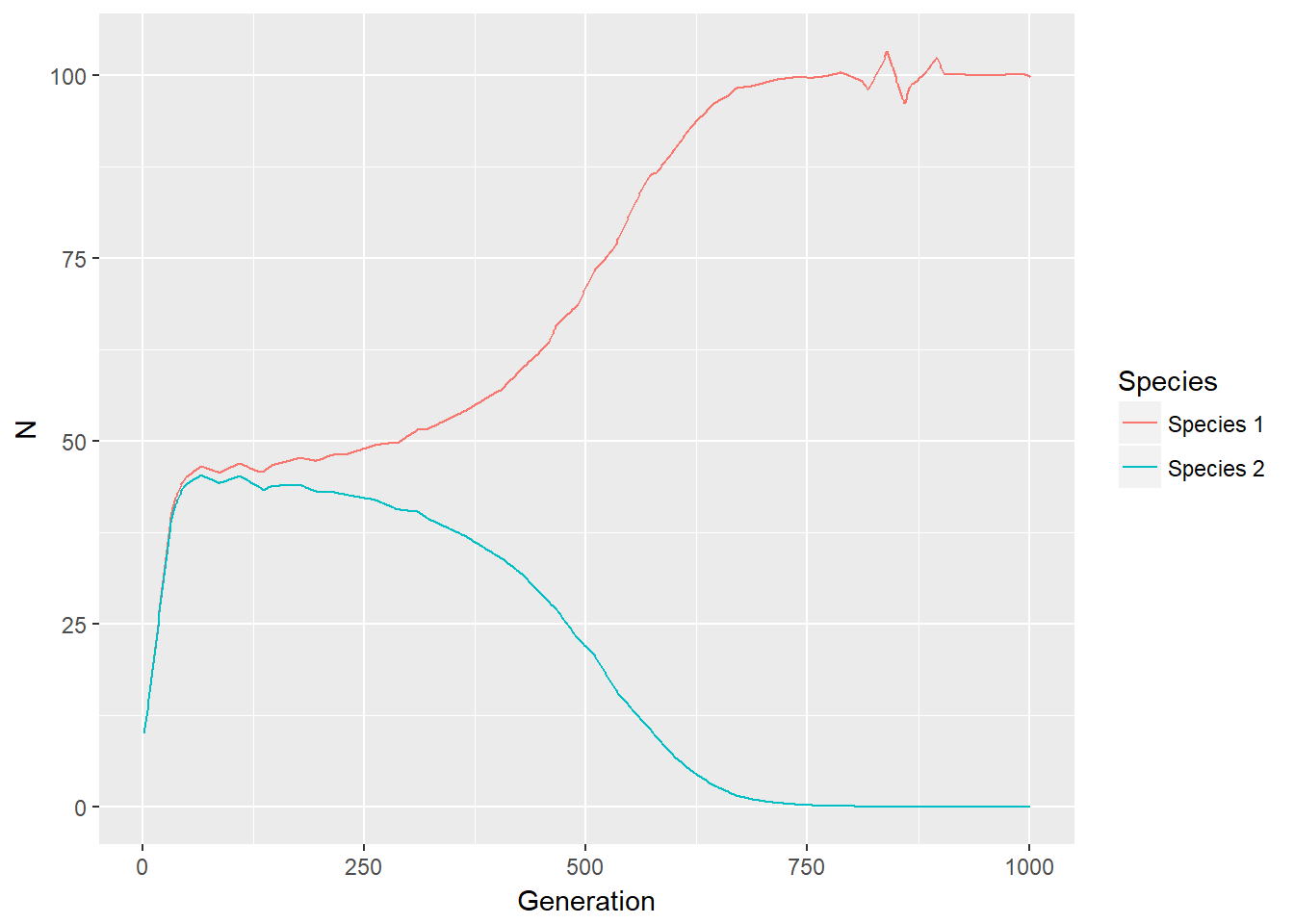### Time series: species 2 lose

Set $$r_1 = 0.1001$$, $$r_2 = 0.1$$

plot_DE(cp_lotka(r1=0.1001,r2=0.1, a12=1.2,a21=1.2, K1=100,K2=100, x1_int=10, x2_int=10))
##  45.45455 45.45455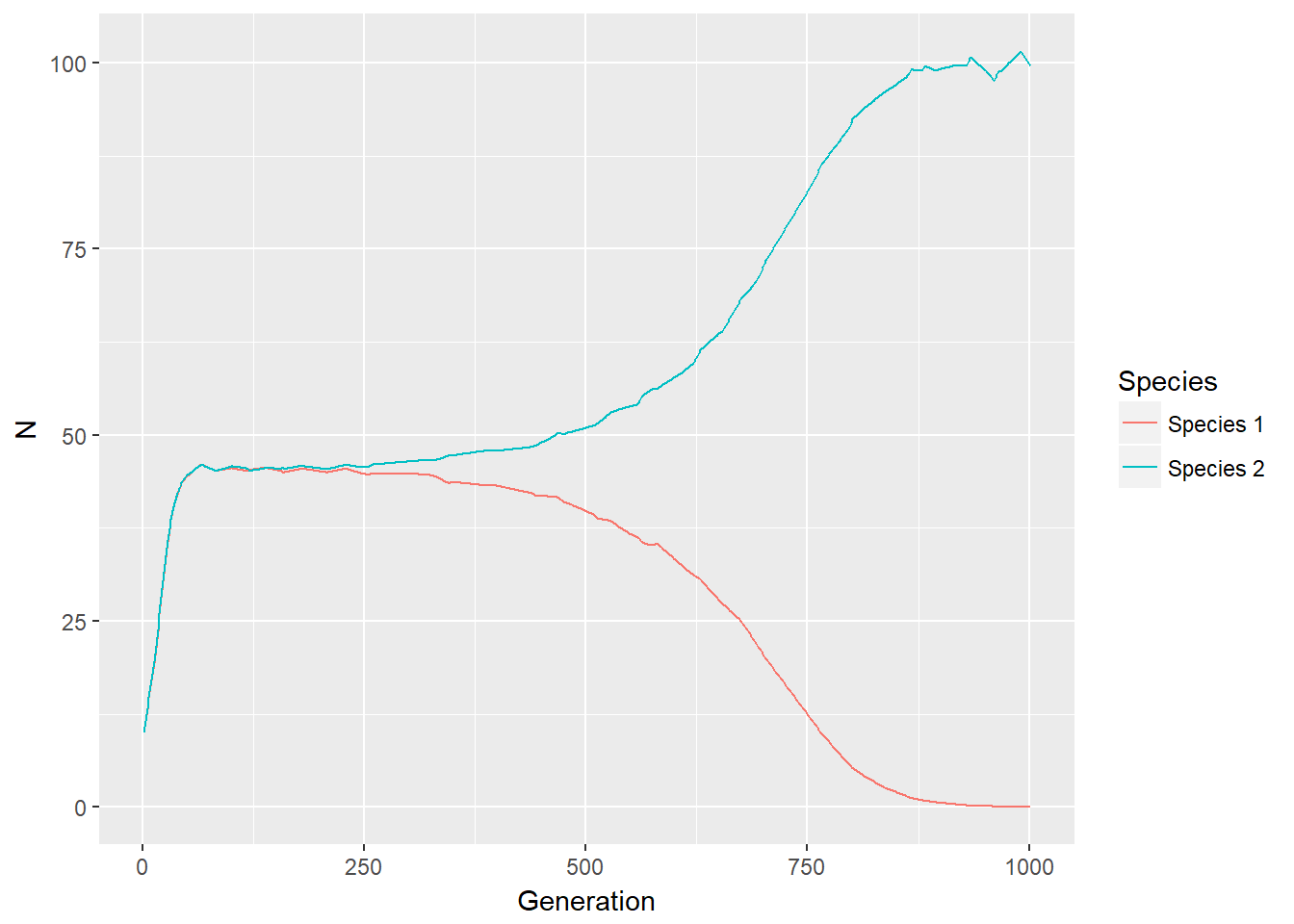### Phase portrait

p <- c(r1=0.1,r2=0.1, a12=1.2,a21=1.2, K1=100,K2=100) # p is a named vector of parameters
s <- c(x1=10,x2=10) # s is the state
plane(tstep=0.5,portrait=T, xmax=150,ymax=150)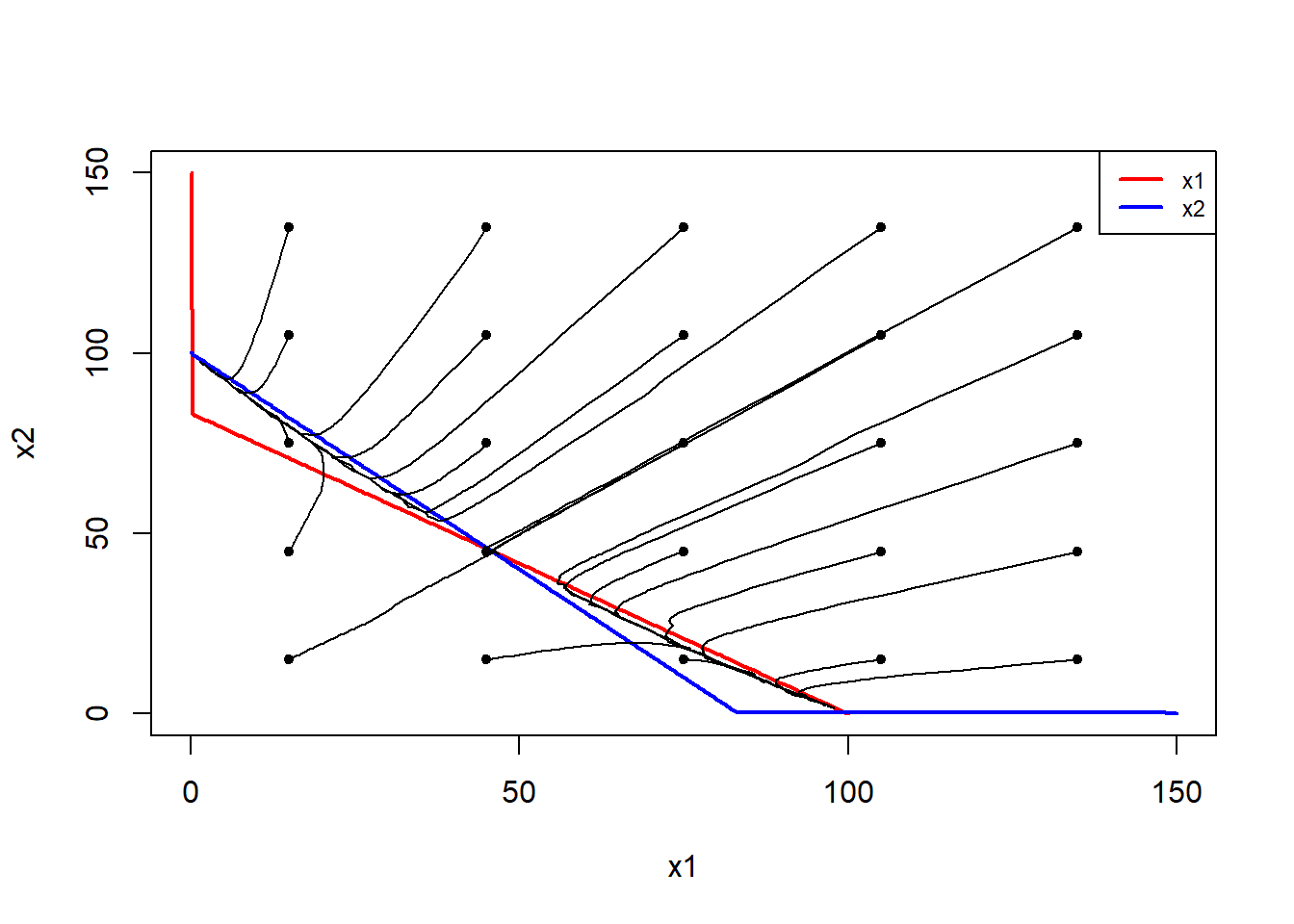## Case 3: Species 2 must lose (with random noises)

$K_1 > \frac{K_2}{\alpha_{21}}$ $K_2 < \frac{K_1}{\alpha_{12}}$

Population size of species 2 has weaker negative impact on the growth rate of species 1 than vice versa.

Set $$K_1=100$$, $$K_2=100$$, $$\alpha_{12}=0.6$$, $$\alpha_{21}=1.2$$,

$$r_1 = 0.1$$, $$r_2 = 0.15$$,

and initial population size: $$x_1=10$$, $$x_2=15$$.

### Time series

plot_DE(cp_lotka(r1=0.1,r2=0.15, a12=0.6,a21=1.2, K1=100,K2=100, x1_int=10, x2_int=15))
##  142.85714 -71.42857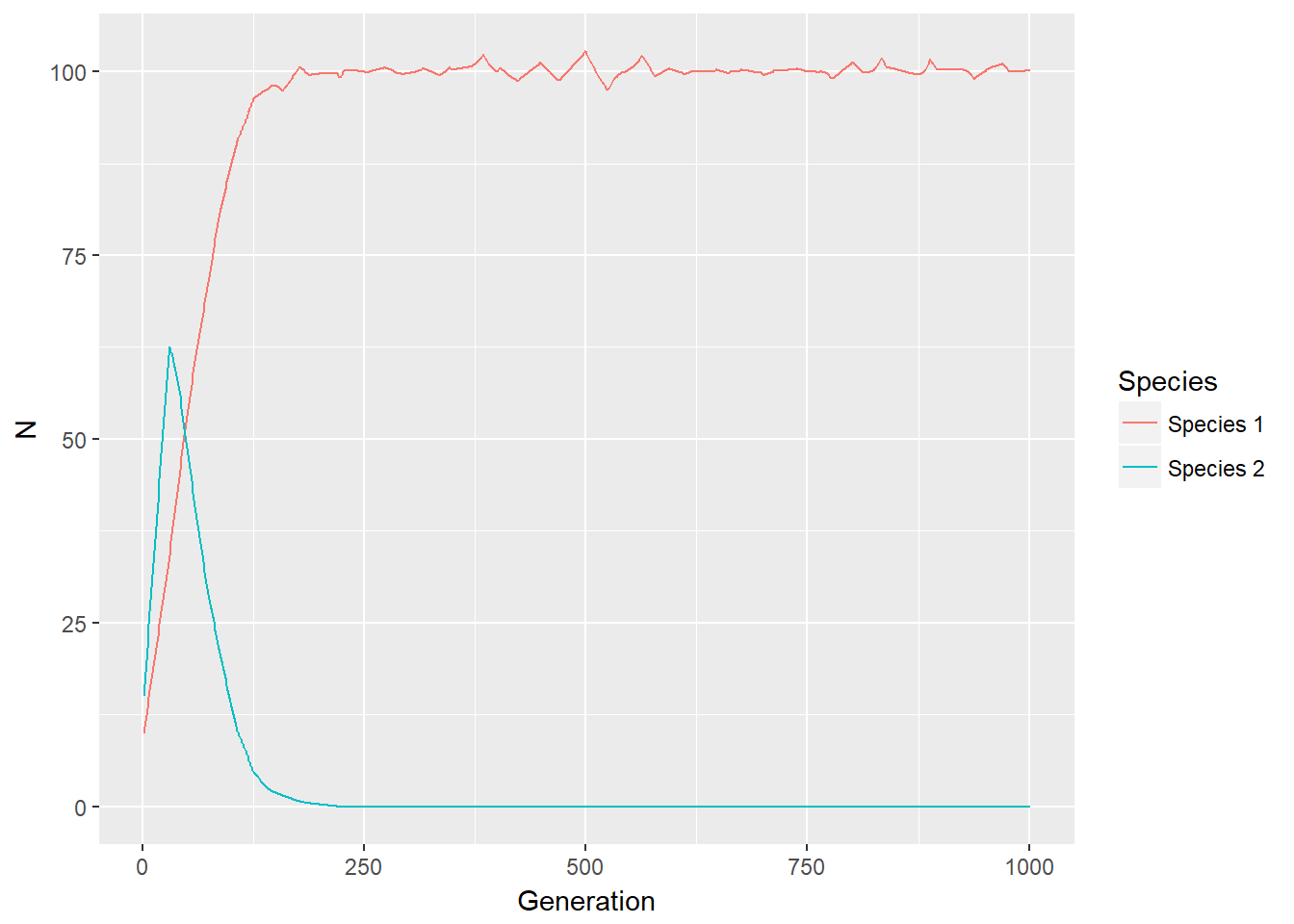### Phase portrait

p <- c(r1=0.1,r2=0.15, a12=0.6,a21=1.2, K1=100,K2=100) # p is a named vector of parameters
s <- c(x1=10,x2=15) # s is the state
plane(tstep=0.5,portrait=T, xmax=150,ymax=150)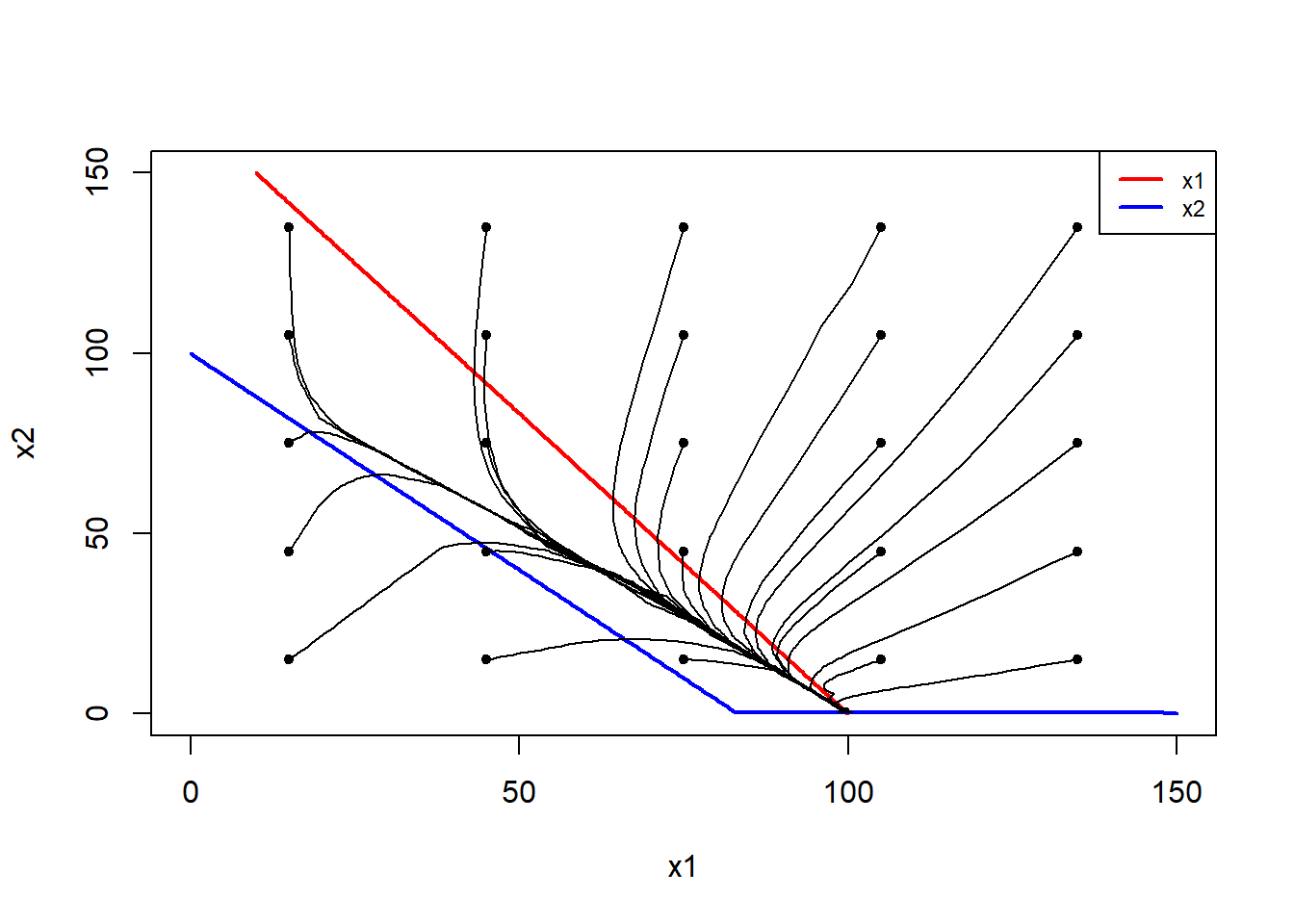## Case 4: Species 1 must lose (with random noises)

$K_1 < \frac{K_2}{\alpha_{21}}$ $K_2 > \frac{K_1}{\alpha_{12}}$

Population size of species 1 has weaker negative impact on the growth rate of species 2 than vice versa.

Set $$K_1=100$$, $$K_2=100$$, $$\alpha_{12}=1.2$$, $$\alpha_{21}=0.6$$,

$$r_1 = 0.3$$, $$r_2 = 0.1$$,

and initial population size: $$x_1=30$$, $$x_2=10$$.

### Time series

plot_DE(cp_lotka(r1=0.3,r2=0.1, a12=1.2,a21=0.6, K1=100,K2=100, x1_int=30, x2_int=10))
##  -71.42857 142.85714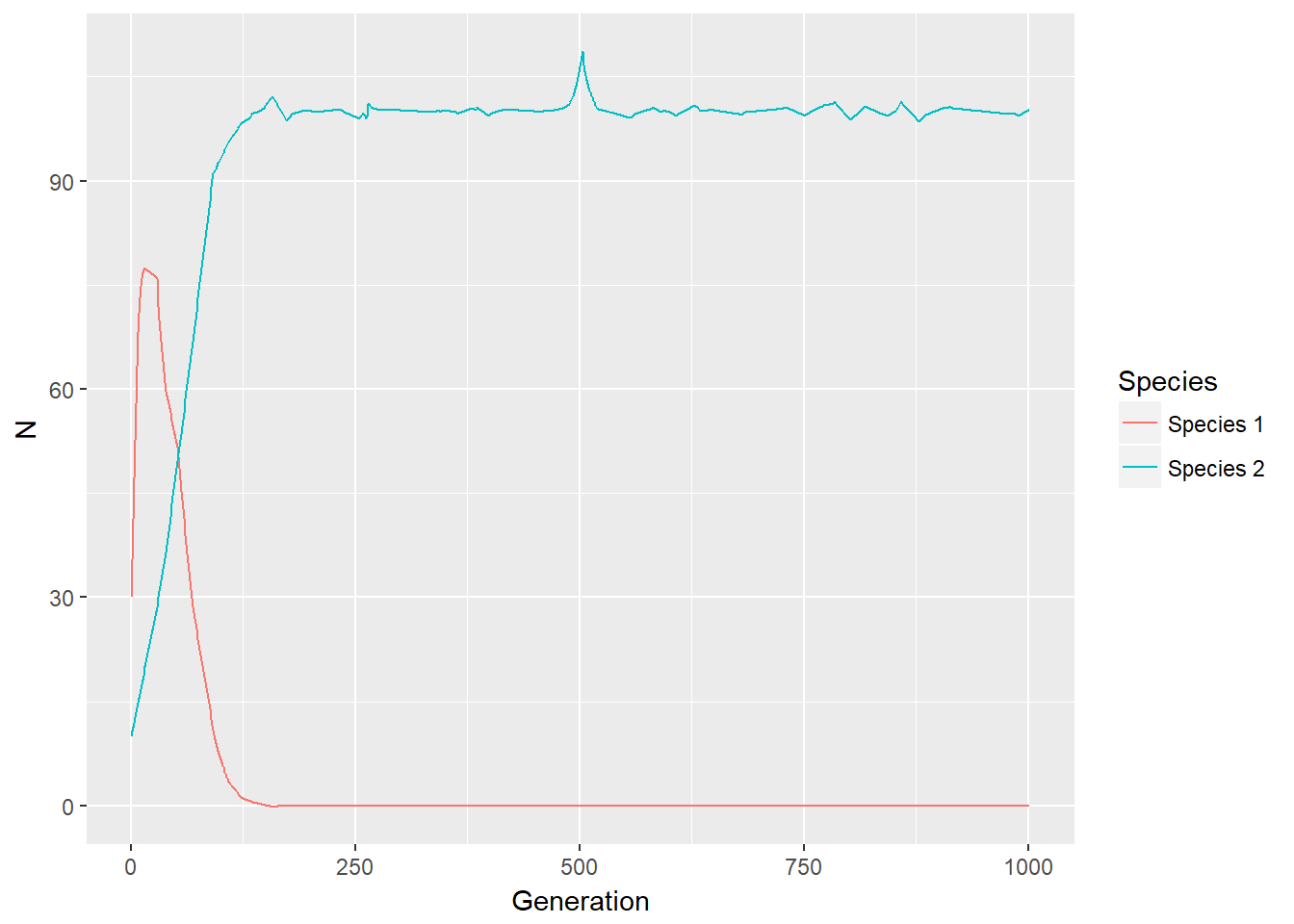### Phase portrait

p <- c(r1=0.3,r2=0.1, a12=1.2,a21=0.6, K1=100,K2=100) # p is a named vector of parameters
s <- c(x1=30,x2=10) # s is the state
plane(tstep=0.5,portrait=T, xmax=150,ymax=150)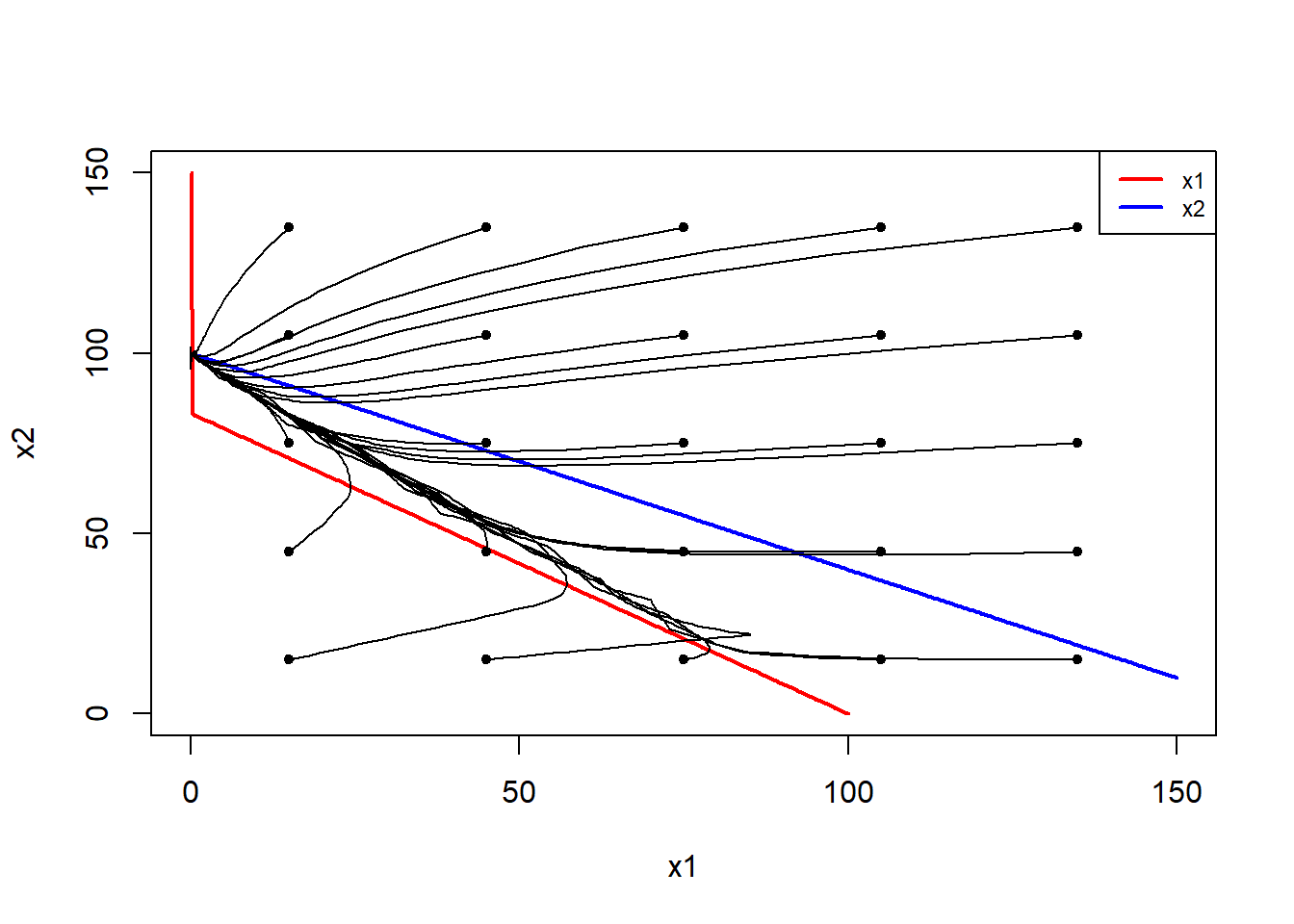## Code

### Function to generate data

cp_lotka <- function(r1,r2,a12,a21,K1,K2,x1_int,x2_int){
library(deSolve)

Pars <- c(r1,r2,a12,a21,K1,K2)
State <- c(x1=x1_int, x2=x2_int)

LotVmod <- function (Time, State, Pars) {
with(as.list(c(State, Pars)), {
dx1 = (1+rnorm(1,0,0.01))*r1*x1*(1-(x1+a12*x2)/(K1+rnorm(1,0,0.05)))   # add random noise: rnorm(1,0,0.01) to replace 0
dx2 = (1+rnorm(1,0,0.01))*r2*x2*(1-(x2+a21*x1)/(K2+rnorm(1,0,0.05)))
return(list(c(dx1, dx2)))
})
}
## equilibrium point
x1 <- (K1-a12*K2)/(1-a12*a21)
x2 <- (K2-a21*K1)/(1-a12*a21)
print(c(x1,x2))

Time <- seq(0, 1000, by = 1)
out <- as.data.frame(ode(func = LotVmod, y = State, parms = Pars, times = Time))
}

### Function to plot 2 species time series

plot_DE <- function(out){
p <- ggplot(out[,-1], aes(x=1:nrow(out)))+
geom_line(aes(y=x1,color="Species 1"))+
geom_line(aes(y=x2,color="Species 2"))
p + labs(x = "Generation",y="N",colour = "Species")
}

### Function for phase portrait

source('PhasePortraitFc.R')

model <- function (Time, State, Pars) {
with(as.list(c(State, Pars)), {
dx1 = (1+rnorm(1,0,0.01))*r1*x1*(1-(x1+a12*x2)/(K1+rnorm(1,0,0.05)))   # add random noise: rnorm(1,0,0.01) to replace 0
dx2 = (1+rnorm(1,0,0.01))*r2*x2*(1-(x2+a21*x1)/(K2+rnorm(1,0,0.05)))
return(list(c(dx1, dx2)))
})
}

PhasePortraitFc.R: credit to Rob de Boer